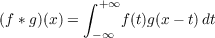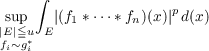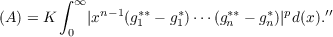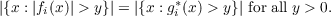#### Vol. 45, No. 1, 1973

 Download this articleFor screen For printingRecent Issues Vol. 325: 1 Vol. 324: 1  2 Vol. 323: 1  2 Vol. 322: 1  2 Vol. 321: 1  2 Vol. 320: 1  2 Vol. 319: 1  2 Vol. 318: 1  2Online Archive Volume: Issue:The Journal Subscriptions Editorial Board Officers Contacts Submission Guidelines Submission Form Policies for Authors ISSN: 1945-5844 (e-only) ISSN: 0030-8730 (print) Special Issues Author Index To Appear Other MSP Journals
Two-sided Lp estimates of convolution transforms

### Gary Sampson

Vol. 45 (1973), No. 1, 347–356
##### Abstract

Let f and g be two Lebesgue measurable functions on the real line. Then the equationdefines the convolution transform of f and g. In an earlier paper  we obtained sharp upper and lower eetimates for the expression(A)

where p = 1,2 and 4, with applications to Fourier transform inequalities. This paper contains estimates of (A) for all values of p(p 1) in the case where E = (−∞,+). For example, one of our theorems implies the following:

“If gi is bounded and has compact support for all i, then there exists a constant K,1(p + 1)p K (p+ 1)p(2n1)p, such thatHere gi are preassigned decreasing functions and the symbol fi gi meansPrimary: 44A35
##### Milestones